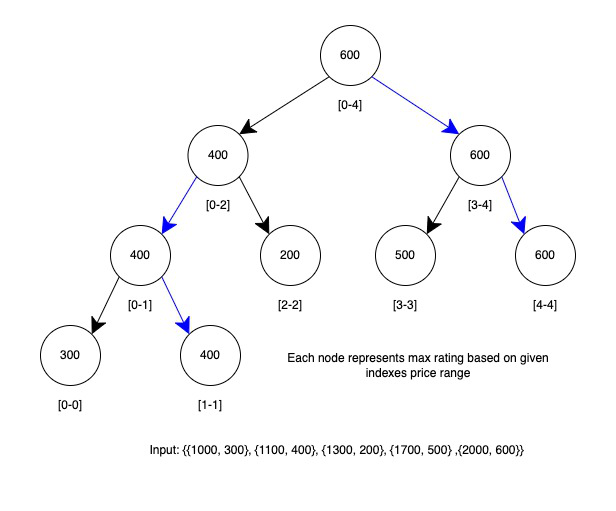# Find element with maximum weight in given price range for Q queries

• Difficulty Level : Expert
• Last Updated : 18 Feb, 2022

Given an array arr[] of size N where each element denotes a pair in the form (price, weight) denoting the price and weight of each item. Given Q queries of the form [X, Y] denoting the price range. The task is to find the element with the highest weight within a given price range for each query.

Examples:

Input: arr[][] = {{24, 6}, {30, 8}, {21, 7}},
queries[][] = {{10, 24}, {20, 30}}
Output: [7, 8]
Explanation: The following are the items chosen for the above range
For first query: There are two items with given range [10, 24] -> {24, 6} and {21, 7} . Highest weight is 7.
For second query: There are two items with given range [20, 30] -> {24, 6}, {21, 7} and {30, 8}. Highest weight is 8.

Input: arr[][] = {{1000, 300}, {1100, 400}, {1300, 200}, {1700, 500}, {2000, 600}},
queries[][] = {{1000, 1400}, {1700, 500}, {2000, 600}}
Output: [400, 500, 600]

Naive Approach: A Simple Solution is to run a loop for the price range and find the maximum weight for each query.

Time Complexity: O(Q*N).
Auxiliary Space: O(1)

Efficient Approach: An efficient approach is to preprocess to store the maximum weight in the price range [i, j] for any i and j. Use Segment Tree for preprocessing and query in moderate time.

Representation of Segment trees

1. Leaf Nodes are the weight corresponding to elements of the input array.
2. Each internal node represents the maximum weight of all leaves under it.

An array representation of a tree is used to represent Segment Trees. For each node at index i, the left child is at index 2*i+1, the right child is at 2*i+2 and the parent is at ⌊(i – 1) / 2⌋.

The solution can be elaborated by dividing the approach into two parts:

1. Construction of Segment Tree from the given array:

• Start with a segment [0 . . . N-1]. and every time divide the current segment into two halves (if it has not yet become a segment of length 1).
• Then call the same procedure on both halves, and for each such segment, store the maximum value in a segment tree node.
Each node here represents the max weight for the given price range between given segment indexes.

Note: All levels of the constructed segment tree will be completely filled except the last level. Also, the tree will be a Full Binary Tree because segments are divided into two halves at every level. Since the constructed tree is always a full binary tree with N leaves.

2. The query for the minimum value of the given range: Once the tree is constructed, how to do range maximum query using the constructed segment tree. Following is the algorithm to get the maximum.

• If the price range of the node is same as the given price range of the query, return the value in the node.
• If the range is completely outside the given range return an extremely high value or say infinite value.
• Otherwise, call a recursive function for both left and right children and return the max received from the recursive calls.

See the image below to understand the formation of segment tree for given input.Image representation of segment tree for the given input

See the following algorithm for better understanding.

// qs –> query start price, qe –> query end price

int RMQ(node, qs, qe)
{
if price range of node is within qs and qe
return value in node
else if price range of node is completely outside qs and qe
return INFINITE
else
return max( RMQ(node’s left child, qs, qe), RMQ(node’s right child, qs, qe) )
}

Below is the implementation of the above approach.

## Java

 `// Java code to implement above approach``import` `java.io.*;``import` `java.util.*;`` ` `class` `GFG {``    ``static` `int``[] segmentTree;`` ` `    ``// Function to get mid``    ``public` `static` `int` `getMid(``int` `start,``                             ``int` `end)``    ``{``        ``return` `start + (end - start) / ``2``;``    ``}`` ` `    ``// Function to fill segment tree``    ``public` `static` `void` `fillSegmentTree(``int``[][] arr)``    ``{``        ``Arrays.sort(arr, ``new` `Comparator<``int``[]>() {``            ``@Override``            ``public` `int` `compare(``int``[] o1,``                               ``int``[] o2)``            ``{``                ``return` `o1[``0``] - o2[``0``];``            ``}``        ``});`` ` `        ``int` `n = arr.length;``        ``int` `maxHeight``            ``= (``int``)Math.ceil(Math.log(n)``                             ``/ Math.log(``2``));``        ``int` `maxSize``            ``= ``2` `* (``int``)Math.pow(``2``, maxHeight) - ``1``;``        ``segmentTree = ``new` `int``[maxSize];`` ` `        ``fillSegmentTreeUtil(segmentTree, arr,``                            ``0``, n - ``1``, ``0``);``    ``}`` ` `    ``// Function to utilise the segment tree``    ``public` `static` `int``    ``fillSegmentTreeUtil(``int``[] segmentTree,``                        ``int``[][] arr,``                        ``int` `start, ``int` `end,``                        ``int` `currNode)``    ``{``        ``if` `(start == end) {``            ``segmentTree[currNode]``                ``= arr[start][``1``];``            ``return` `segmentTree[currNode];``        ``}`` ` `        ``int` `mid = getMid(start, end);``        ``segmentTree[currNode] = Math.max(``            ``fillSegmentTreeUtil(segmentTree,``                                ``arr, start,``                                ``mid, currNode``                                             ``* ``2``                                         ``+ ``1``),``            ``fillSegmentTreeUtil(segmentTree,``                                ``arr, mid + ``1``,``                                ``end, currNode``                                             ``* ``2``                                         ``+ ``2``));``        ``return` `segmentTree[currNode];``    ``}`` ` `    ``// Function to find the maximum rating``    ``public` `static` `int` `findMaxRating(``int``[][] arr,``                                    ``int``[] query)``    ``{``        ``int` `n = arr.length;``        ``return` `findMaxRatingUtil(segmentTree,``                                 ``arr, ``0``, n - ``1``,``                                 ``query[``0``],``                                 ``query[``1``], ``0``);``    ``}`` ` `    ``// Function to utilise the``    ``// maxRating function``    ``public` `static` `int``    ``findMaxRatingUtil(``int``[] segmentTree,``                      ``int``[][] arr,``                      ``int` `start, ``int` `end,``                      ``int` `qStart,``                      ``int` `qEnd, ``int` `currNode)``    ``{``        ``if` `(qStart <= arr[start][``0``]``            ``&& qEnd >= arr[end][``0``]) {``            ``return` `segmentTree[currNode];``        ``}``        ``if` `(qStart > arr[end][``0``] || qEnd < arr[start][``0``]) {``            ``return` `-``1``;``        ``}``        ``int` `mid = getMid(start, end);``        ``return` `Math.max(``            ``findMaxRatingUtil(segmentTree,``                              ``arr, start, mid,``                              ``qStart, qEnd,``                              ``currNode * ``2` `+ ``1``),``            ``findMaxRatingUtil(segmentTree,``                              ``arr, mid + ``1``,``                              ``end, qStart, qEnd,``                              ``currNode * ``2` `+ ``2``));``    ``}`` ` `    ``// Driver code``    ``public` `static` `void` `main(String[] args)``    ``{``        ``int``[][] arr = { { ``1000``, ``300` `},``                        ``{ ``1100``, ``400` `},``                        ``{ ``1300``, ``200` `},``                        ``{ ``1700``, ``500` `},``                        ``{ ``2000``, ``600` `} };`` ` `        ``fillSegmentTree(arr);`` ` `        ``int``[][] queries = { { ``1000``, ``1400` `},``                            ``{ ``1700``, ``1900` `},``                            ``{ ``0``, ``3000` `} };`` ` `        ``for` `(``int``[] query : queries) {``            ``System.out.println(``                ``findMaxRating(arr, query));``        ``}``    ``}``}`
Output
```400
500
600
```

Time Complexity: O(N log N + Q*log N)
Auxiliary Space: O(N)

Analyzing Time Complexity: Time Complexity for sorting the given input array is O(N * LogN)
Time Complexity for tree construction is O(N). There is a total of 2N-1 nodes, and the value of every node is calculated only once in tree construction.
The time complexity for each query is O(LogN). To query a range maximum, process at most two nodes at every level, and the number of levels is O(LogN)

My Personal Notes arrow_drop_up